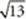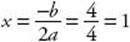﻿ ﻿CIRCLES AND PARABOLAS - Coordinate Geometry - SOLID AND COORDINATE GEOMETRY - SAT SUBJECT TEST MATH LEVEL 1

## SOLID AND COORDINATE GEOMETRY## CHAPTER 13Coordinate Geometry### CIRCLES AND PARABOLAS

Besides the line, you need to know the equations for two other geometric shapes for the Math 1 test: the circle and the parabola.

Chapter 11 discussed all the facts you need to know about circles. In this chapter, we review the standard equation of a circle, which is given in KEY FACT L10.

Key Fact L10

The equation of the circle whose center is the point (h, k) and whose radius is r is (xh)2 + (yk)2 = r 2.If the center is at the origin, (0, 0), then h = k = 0 and the equation reduces to x2+ y2= r2.To write the equation of a circle, you need to know its center and its radius. Either they will be specifically given to you or you will be given some other information that will enable you to determine them.

EXAMPLE 11: What is the equation of the circle whose center is at (–3, 2) and whose radius is 5?

Plug h = –3, k = 2, and r = 5 into the standard equation:EXAMPLE 12: In Examples 3 and 4, we considered the circle in which C(3, –4) and D(7, 2) are the endpoints of a diameter. What is the equation of this circle?

In Example 3, we used the midpoint formula to find that the center is at (5, –1). In Example 4, we used the distance formula to get that the radius is. Therefore, the equation for this circle is:There are many facts about parabolas that you do not need for the Math 1 test. In particular, you do not need to know the precise definition of parabola in terms of its focus and directrix. Basically, you need to know the general equation of a parabola and to recognize that the graph of a parabola is a U-shaped curve that is symmetrical about a line, called the axis of symmetry, which passes through the parabola”s vertex, or turning point. Any parabola you see on a Math 1 test will likely have a vertical axis of symmetry.KEY FACT L11 lists the equations you need to know about parabolas.

Key Fact L11

For any real numbers a, b, c with a0:

y = ax2+ bx + c is the equation of a parabola whose axis of symmetry is a vertical line.

Conversely, the equation of any parabola with a vertical axis of symmetry has an equation of the form y = ax2 + bx + c.

The equation of the parabola”s axis of symmetry is.

The vertex of the parabola is the point on the parabola whose x-coordinate is.

If a > 0, the parabola opens upward and the vertex is the lowest point on the parabola.

If a < 0, the parabola opens downward and the vertex is the highest point on the parabola.EXAMPLE 13: To determine the vertex (turning point) of the parabola y = 2x 2 – 4x + 5, first find its axis of symmetry. Since a = 2, b = –4, and c = 5, the equation of the axis of symmetry is. So the x-coordinate of the turning point is 1 and the y-coordinate is

2(1)2 – 4(1) + 5 = 2 – 4 + 5 = 3

The turning point is (1, 3).

Of course, an alternative solution would be to graph y = 2x 2 – 4x + 5 on a graphing calculator and to trace along the parabola until the cursor is at the turning point.

Key Fact L12

Given any three points, P, Q, and R that do not lie on a line, there is a parabola with a vertical axis of symmetry that passes through them.

EXAMPLE 14: To find the equation of a parabola that passes through (0, 4), (1, 3), and (2, 6), plug the x and y coordinates of the points into the equation y = ax2 + bx + c.Subtract these last two equations:Then by replacing a by 2 in the equation a + b = –1, we get that b = –3.

So the equation of the parabola that passes through the three given points is y = 2x 2 – 3x + 4.

There is an important relationship between the parabola y = ax 2 + bx + c and the quadratic equation ax 2 + bx + c = 0.

Key Fact L13

The x-intercepts of the graph of the parabola y = ax2+ bx + c are the (real) solutions of the equation ax 2 + bx + c = 0.

Consider the graphs of the following six parabolas.

(i)(ii)(iii)(iv)(v)(vi)I. Since the graph of y = x 2 – 6x + 8 crosses the x–axis at 2 and 4, the quadratic equation x 2 – 6x + 8 = 0 has two real solutions: x = 2 and x = 4.

II. Since the graph of y = 2x 2 – 8x + 8 crosses the x-axis only at 2, the quadratic equation 2x 2 – 8x + 8 = 0 has only one real solution: x = 2.

III. Since the graph of y = 2x 2 – 8x + 9 does not cross the x-axis, the quadratic equation 2x 2 – 8x + 9 = 0 has no real solutions.

Similarly, from graphs iv, v, and vi, you can see that the equation –x 2 + 4 = 0 has two real solutions; the equation –x 2 + 6x – 9 = 0 has only one real solution; and the equation –x 2 + 6x – 11 = 0 has no real solutions.

﻿